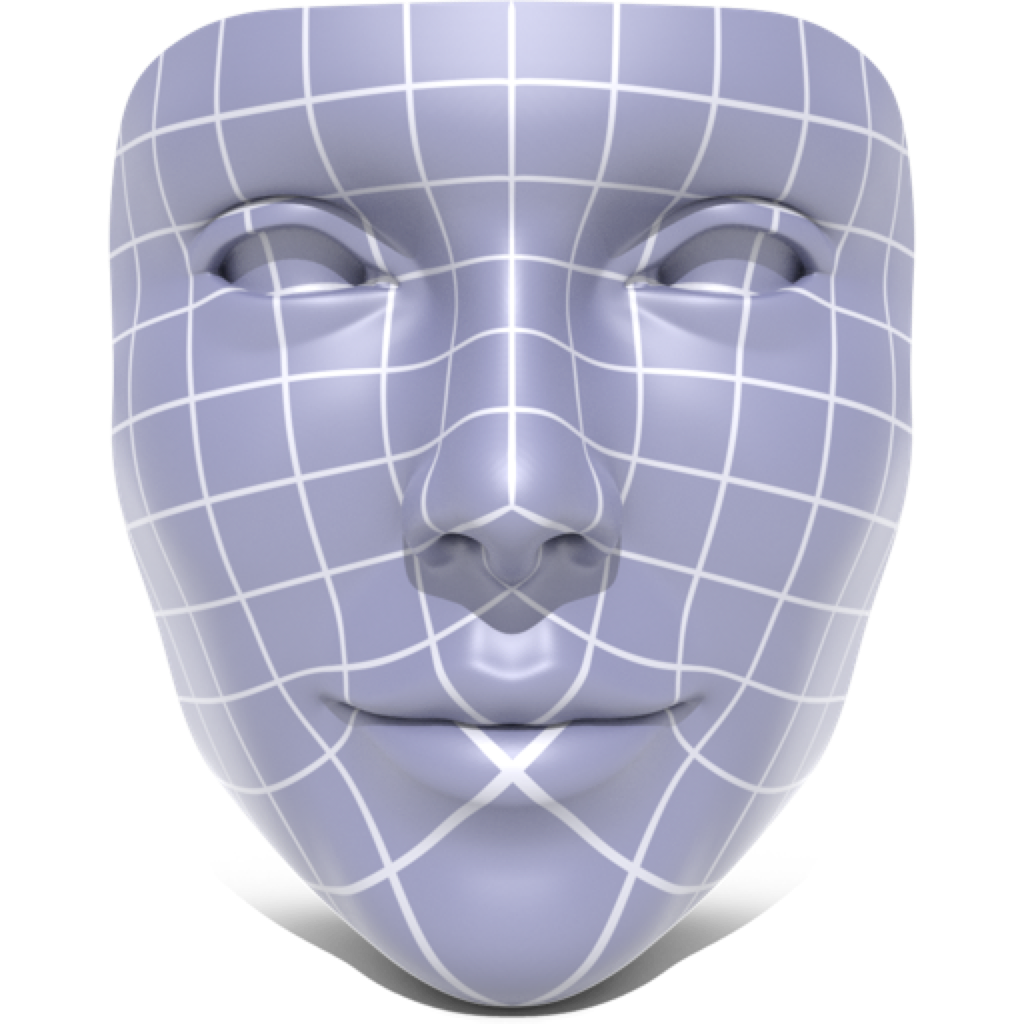Parameterization

This section describes the algorithms in geometry-central for surface parameterization, which compute maps from surfaces meshes to the plane.

Note that these procedures all depend on the intrinsic geometry of a surface (via the IntrinsicGeometryInterface). Therefore, these routines can be run on abstract geometric domains as well as traditional surfaces in 3D.

Boundary First FlatteningThis algorithm is described in the paper Boundary First Flattening. It computes a conformal parameterization of a surface mesh, allowing the user to specify target angles or scale factors along the boundary of the mesh. The input mesh must be a topological disk.

#include "geometrycentral/surface/boundary_first_flattening.h"

Single Parameterizations

A one-off utility function is provided to compute single parameterizations. Repeated parameterizations of the same mesh can be computed more efficiently using the utility class BFF below.

Example:

#include "geometrycentral/surface/boundary_first_flattening.h"
#include "geometrycentral/surface/meshio.h"

std::unique_ptr<ManifoldSurfaceMesh> mesh;
std::unique_ptr<VertexPositionGeometry> geometry;

VertexData<Vector2> parameterization = parameterizeBFF(*mesh, *geometry);

VertexData<Vector2> parameterizeBFF(ManifoldSurfaceMesh& mesh, IntrinsicGeometryInterface& geom)

Conformally parameterize the input mesh. Picks boundary conditions to minimize area distortion (i.e. sets the conformal scale factor to 0 along the boundary).

VertexData<Vector2> parameterizeBFFfromScaleFactors(ManifoldSurfaceMesh& mesh, IntrinsicGeometryInterface& geom, const VertexData<double>& boundaryScaleFactors)

Conformally parameterize the input mesh, setting the scale factors to the given values along the boundary. Although boundaryScaleFactors is a VertexData object which stores values at all vertices, only the values at boundary vertices are used by the algorithm. All other values are ignored.

VertexData<Vector2> parameterizeBFFfromExteriorAngles(ManifoldSurfaceMesh& mesh, IntrinsicGeometryInterface& geom, const VertexData<double>& exteriorAngles)

Conformally parameterize the input mesh so that the boundary vertices of the parameterized mesh have the given exterior angles. The exterior angles must sum up to $2\pi$ along the boundary.

Although exteriorAngles is a VertexData object which stores values at all vertices, only the values at boundary vertices are used by the algorithm. All other values are ignored.

Repeated Parameterization

The stateful class BFF does precomputation when constructed to efficiently compute many parameterizations of the same mesh.

Example:

#include "geometrycentral/surface/boundary_first_flattening.h"
#include "geometrycentral/surface/meshio.h"

std::unique_ptr<ManifoldSurfaceMesh> mesh;
std::unique_ptr<VertexPositionGeometry> geometry;

VertexData<double> boundaryScaleFactors = /* target scale factors */;
VertexData<double> exteriorAngles = /* target exteriorAngles */;

BFF bff(*mesh, *geometry);
VertexData<Vector2> parameterization1 = bff.flattenFromScaleFactors(boundaryScaleFactors);
VertexData<Vector2> parameterization2 = bff.flattenFromExteriorAngles(exteriorAngles);

BFF::BFF(ManifoldSurfaceMesh& mesh, IntrinsicGeometryInterface& geom)

Create a new solver for boundary first flattening. Most precomputation is done immediately when the object is constructed, although some additional precomputation may be done lazily later on.

VertexData<Vector2> BFF::flatten()

Compute a conformal parameterization which minimizes area distortion (i.e. sets the scale factor to 0 along the boundary).

VertexData<Vector2> BFF::flattenFromScaleFactors(const VertexData<double>& boundaryScaleFactors)

Compute a conformal parameterization with the given scale factor along the boundary. Although boundaryScaleFactors is a VertexData object which stores values at all vertices, only the values at boundary vertices are used by the algorithm. All other values are ignored.

VertexData<Vector2> BFF::flattenFromExteriorAngles(const VertexData<double>& exteriorAngles)

Compute a conformal parameterization with the given exterior angles along the boundary. The exterior angles must sum up to $2\pi$ along the boundary.

Although exteriorAngles is a VertexData object which stores values at all vertices, only the values at boundary vertices are used by the algorithm. All other values are ignored.

Citation

If this algorithm contributes to academic work, please cite the following paper:

@article{Sawhney:2017:BFF,
author = {Sawhney, Rohan and Crane, Keenan},
title = {Boundary First Flattening},
journal = {ACM Transactions on Graphics (TOG)},
volume = {37},
number = {1},
month = dec,
year = {2017},
issn = {0730-0301},
pages = {5:1--5:14},
articleno = {5},
numpages = {14},
url = {http://doi.acm.org/10.1145/3132705},
doi = {10.1145/3132705},
acmid = {3132705},
publisher = {ACM},
address = {New York, NY, USA}
}